# SBI PO Reasoning Questions 2019 (Day-34) High Level-New Pattern

Dear Aspirants, the most awaited notification of SBI PO – 2019 has been released. We all know that new pattern questions are introducing every year in the SBI PO exam. Further, the questions are getting tougher and beyond the level of the candidate’s expectations.

Our IBPS Guide is providing High-Level New Pattern Reasoning Ability Questions for SBI PO 2019 so the aspirants can practice it on a daily basis. These questions are framed by our skilled experts after understanding your needs thoroughly. Aspirants can practice these high-level questions daily to familiarize with the exact exam pattern. We wish that your rigorous preparation leads you to a successful target of becoming SBI PO.

[WpProQuiz 5825]

### Click Here for SBI PO Pre 2019 High-Quality Mocks Exactly on SBI Standard

(Directions 1–5): A series of four digit number is given as input. The further steps given are obtained by applying certain logic. Each step is a resultant of previous step only. Study the following information carefully and answer the questions given below it.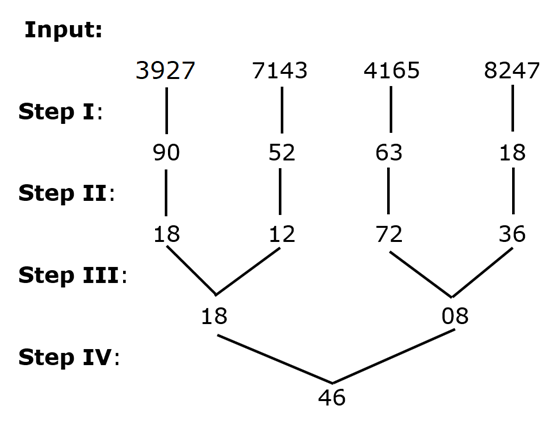As per above applied logic in above steps, find appropriate step for given input: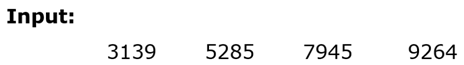1) What is the sum of digits of second lowest number formed in step II?

a) 13

b) 3

c) 7

d) 11

e) None of these

2) Which of the following number represents final output from step IV?

a) 13

b) 32

c) 7

d) 30

e) None of these

3) Which of the product of sum of digits of second highest and second lowest number from step I?

a) 42

b) 84

c) 96

d) 63

e) None of these

4) Which is the difference of numbers formed in step III?

a) 292

b) 164

c) 72

d) 148

e) None of these

5) Which is the sum of numbers formed in step II?

a) 175

b) 142

c) 156

d) 133

e) None of these

Directions (6-10): Study the following information carefully and answer the below questions.

Sixteen students from class 5th and 6th namely – Monty, Sonu, Sam, Tom, Dev, Sonty, Deep, Krish, Hena, Monu, Paul, Hari, Smith, Madhu, Nitya and Ritu are sitting in two parallel rows in such a way that the one who belongs to 5th class sits facing south and the one who belongs to 6th class sits facing north. Each person also belongs to four different cultural group viz. Tagore (T), Gandhi (G), Patel (P) and Nehru (N). No two student from same group either sits adjacent or facing each other. Not more than two students from same group sits in either rows. Weight of each student is also different viz. 42, 44, 48, 52, 54, 56, 60, 62, 68, 72, 76, 80, 84, 90, 92 and 96 kg. All the information are not necessary in same order.

Paul who belongs to group N sits third to right of the one whose weight is 80kg. The one whose weight is 56kg sits immediate right of Monu. Two person sits between the one whose weight is 60kg and Deep, who neither belongs to group G nor sits at end of the row. Madhu sits facing the one whose weight is 76kg and sits at a gap of two person from the one whose weight is 42kg. The one whose weight is 62kg sits facing the one who sits adjacent to the one whose weight is 80kg. Three person sits between the one whose weight is 62kg and Hena, who belongs to group P. At least four person sits between the one whose weight is 68kg and the one whose weight is 44kg. Smith sits second from left end and belongs to group T. The one whose weight is 72kg sits at a gap of two person from the one whose weight is 84kg. Two person sits between Nitya and the one who sits facing the one whose weight is 52kg. Weight of Nitya is neither 60kg nor 80kg. The one whose weight is 90kg belongs to group N and sits facing Monty, who belongs to 5th class. The one whose weight is 60kg sits adjacent to Hena but neither belongs to group G nor group N. Ritu belongs to group T but her weight is not more than 75kg. Two person sits between Smith and the one whose weight is 54kg. One person sits between Tom and Sonu, whose weight is neither 54kg nor belongs to group N. The one whose weight is 54kg sits adjacent to Paul, whose weight is neither 72kg nor 90kg. The one whose weight is 90kg neither sits adjacent of Hena nor adjacent of Deep. Hari sits second to right of Sonty, whose weight is neither 96kg nor belongs to group G. Three person sits between Monty and Dev, whose weight is 52kg but doesn’t sit at end of the row. The one whose weight is 42kg belongs to group P and sits immediate right of the one who belongs to group G. Tom sits facing Sam, whose weight is neither 68kg nor belongs to group N. Three person sits between Sonu and the one whose weight is 56kg. At least two person sits between the one whose weight is 96kg and Krish. The one whose weight is 44kg sits facing the one who belongs to group G. The one whose weight is 92kg sits adjacent of the one who sits facing the one who belongs to group P. One person sits between the one whose weight is 48kg and the one who belongs to group P.

6) What is the position of the one whose weight is 44kg with respect to Madhu?

a) Third to right

b) Fourth to right

c) second to left

d) Immediate right

e) None of these

7) Which of the following pair of students belong to group G from 6th class?

a) Sonu and Nitya

b) Sam and Sonu

c) Hari and Sam

d) Monu and Nitya

e) None of these

8) Which of the following statement is not true?

a) The one whose weight is 92kg sits immediate right of the one who sits facing Krish.

b) Hena sits facing the one who sits immediate right of the one who belongs to group T.

c) Tom sits fifth to right of the one whose weight is 62kg.

d) Dev sits facing the one who sits second to left of Monu.

e) More than one statement is false.

9) How many person sits between Sonu and the one who sits facing Smith?

a) Two

b) Four

c) One

d) Three

e) None

10) Which of the following combination is not true?

a) Monty – 42 – Patel

b) Paul – 68 – Nehru

c) Ritu – 72 – Gandhi

d) Hena – 84 – Patel

e) Nitya – 56 – Gandhi

Directions (1-5):

We have: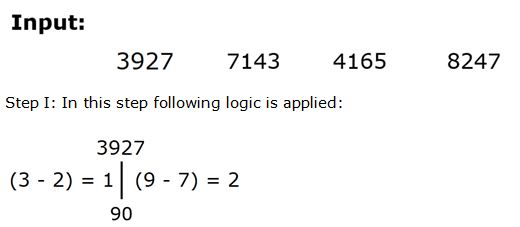Clearly, result in step I can be obtained by square of sum of digits obtained.

Result = (2 + 1)2 = 9

Then, final result can be obtained by interchanging the digits.

Thus, final result in the step I after interchanging ‘9’ à 90

Step II: In this step following logic is applied: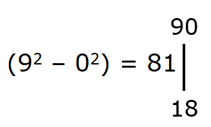Clearly, result in step II can be determined by difference of square of digits.

Result = (92 – 02) = 81

Then, final result in step II can be obtained by interchanging digits of result.

Thus, final result from ‘81’ à 18

Step III: In this step following logic is applied: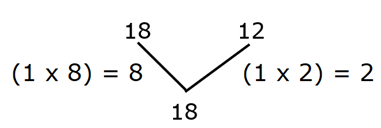Clearly, result in step III can be determined by difference of product of digits from step I:

Result = (1 x 8) – (1 x 2) = 6

Then, final result in step III can be obtained by dividing square of digit by ‘2’.

Thus, final result = 62/2 = 18.

Step IV: In this step following logic is applied: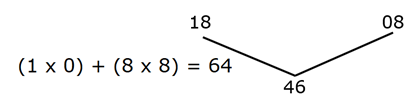Clearly, results in step IV can be determined by sum of products of digits.

Result = (1 x 0) + (8 x 8) = 64

Then, final result in step IV can be determined by interchanging digits of results.

Thus, final result from ‘64’ -> 46

Thus, from above given steps we final following result for given input: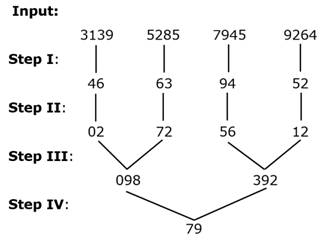Clearly, sum of digits of second highest number in step II à (1 + 2) = 3

Hence, option B is correct choice.

Clearly, ‘79’ is the final output from step IV.

Hence, option E is correct choice.

Clearly, second highest number from step I à (6 + 3) = 9

Second lowest number from step I à (5 + 2) = 7

Required product = (9 x 7) = 63

Hence, option D is correct choice.

Clearly, required difference = (392 – 98) = 294

Hence, option E is correct choice.

Clearly, sum of numbers = (02+72+56+12)= 142

Hence, option B is correct choice.

Direction (6-10) :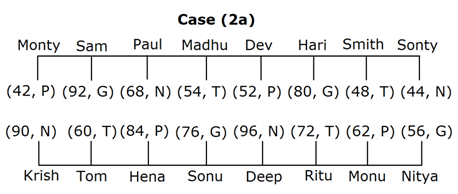We have:

• Smith sits second from left end and belongs to group T, that we have two possible place for Smith, in case (1) Smith sits facing north and in case (2) Smith sits facing south.
• Two person sits between Smith and the one whose weight is 54kg.
• The one whose weight is 54kg and Paul sits together, that means in case (1a) & case (2a) Paul sits immediate right of the one whose weight is 54kg, in case (1b) & case (2b) Paul sits immediate left of the one whose weight is 54kg.
• Paul who belongs to group N sits third to right of the one whose weight is 80kg.

Based on above given information we have: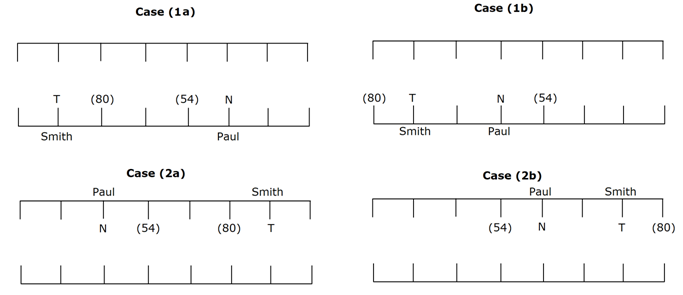Again, we have:

• The one whose weight is 62kg sits facing the one who sits adjacent to the one whose weight is 80kg, that means in case (1a), case (1b), case (2a), case (2b) the one whose weight is 62kg sits facing Smith, in case (1c) & case (2c) the one whose weight is 62kg sits fourth from right end.
• Three person sits between the one whose weight is 62kg and Hena, who belongs to group P.
• The one whose weight is 60kg sits adjacent to Hena but neither belongs to group G nor group N, as no two students from same group sits adjacent, that means in case (1a), case (1b), case (2a) & case (2b) the one whose weight is 60kg sits immediate left of Hena and belongs to group T, in case (1c), case (1d), case (1e), case (2c), case (2d) & case (2e) the one whose weight is 60kg sits immediate right of Hena and belongs to group T.
• Two person sits between the one whose weight is 60kg and Deep, who neither belongs to group G nor sits at end of the row.
• The one whose weight is 90kg neither sits adjacent of Hena nor adjacent of Deep.
• The one whose weight is 90kg belongs to group N and sits facing Monty, who belongs to 5th
• Three person sits between Monty and Dev, whose weight is 52kg but doesn’t sit at end of the row, that means in case (1a), case (1c), case (2a) & case (2d) Monty sits at right end, in case (1b) Monty sits from second from left end, in case (1d) & case (1e) Monty sits at left end, in case (1f) Monty sits second from left end and case (2b), case (2c) & case (2e) are not valid.

Based on above given information we have: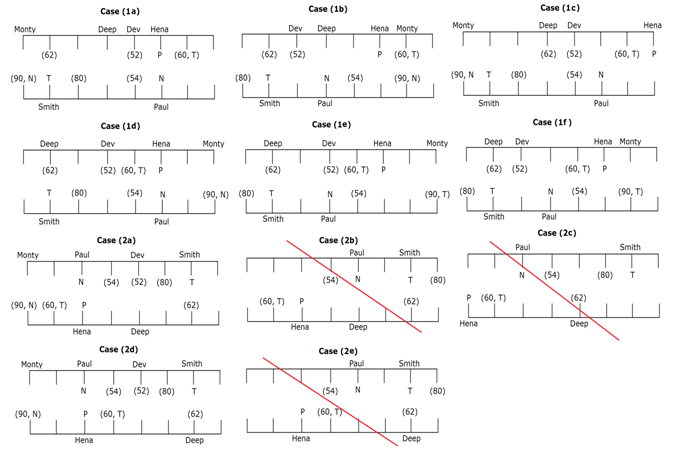Case (2b), case (2c) & case (2e) are not valid as three person sits between Monty and Dev.

Again, we have:

• Two person sits between Nitya and the one who sits facing the one whose weight is 52kg.
• Weight of Nitya is neither 60kg nor 80kg, that means in case (1a), case (1c), case (2a), case (2f) Nitya sits at right end facing north, in case (1b), case (1e), case (1f), case (1g) Nitya sits second from right end facing north, in case (1d) Nitya sits at left end facing north, in case (2d) Nitya sits second from left end facing north.
• Tom sits facing Sam, whose weight is neither 68kg nor belongs to group N.
• One person sits between Tom and Sonu, whose weight is neither 54kg nor belongs to group N.
• Three person sits between Sonu and the one whose weight is 56kg.
• The one whose weight is 56kg sits immediate right of Monu, that means in case (1d) Tom sits at left end and sits facing north, in case (1h) Tom sits fourth from right end and sits facing north, in case (2a) Tom sits second from left end and sits facing north, in case (2d), case (2f) & case (2i) Tom sits fourth from left end and sits facing north, in case (2g) Tom sits third from right end and sits facing north, in case (2h) Tom sits at right end and sits facing north, and case (1a), case (1b), case (1c), case (1d), case (1f) and case (1g) are not valid.

Based on above given information we have: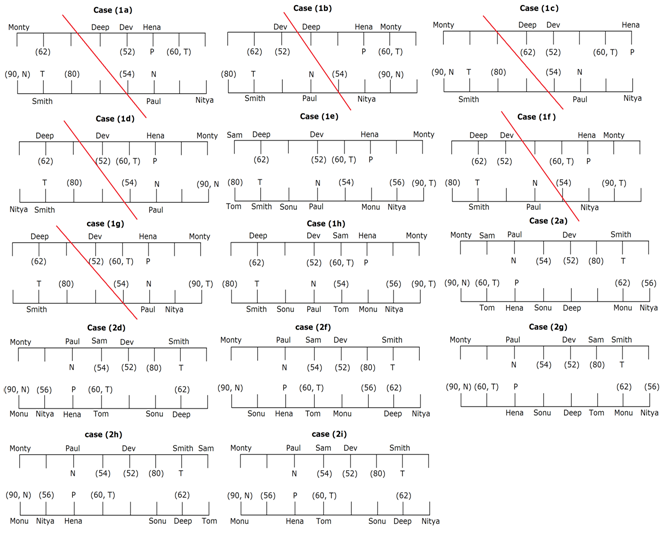Case (1a), case (1b), case (1c), case (1d), case (1f) and case (1g) are not valid as The one whose weight is 56kg sits immediate right of Monu.

Again, we have:

• Madhu sits facing the one whose weight is 76kg and sits at a gap of two person from the one whose weight is 42kg.
• The one whose weight is 42kg belongs to group P and sits immediate right of the one who belongs to group G, as Deep doesn’t belong to group G, that means in case (1e) & case (1h) Madhu sits third from right end and sits facing south, in case (2a) & case (2g) Madhu sits fourth from right end and sits facing south and case (2d), case (2f), case (2h) & case (2i) are not valid.
• The one whose weight is 72kg sits at a gap of two person from the one whose weight is 84kg, that means in case (2a) & case (2g) weight of Hena is 84kg, in case (2j) & case (2k) weight of Hena is 72kg and case (1e) & case (1h) are not valid.

Based on above given information we have: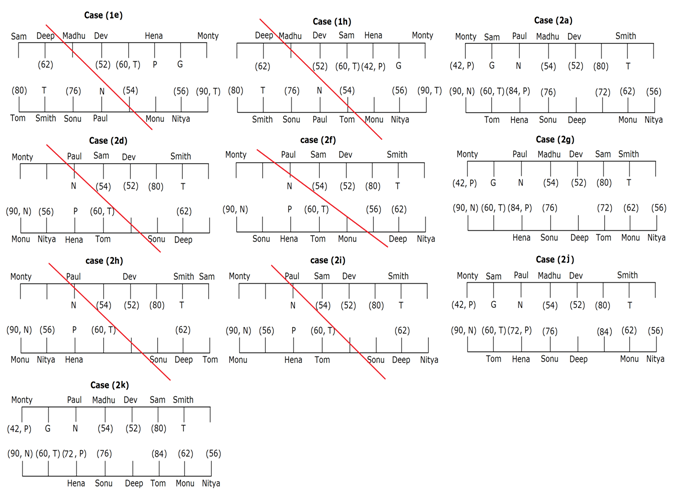Case (2d), case (2f), case (2h) & case (2i) are not valid as the one whose weight is 42kg belongs to group P and sits immediate right of the one who belongs to group G and case (1e) & case (1h) are not valid are not valid as The one whose weight is 72kg sits at a gap of two person from the one whose weight is 84kg.

Again, we have:

• Hari sits second to right of Sonty, whose weight is neither 96kg nor belongs to group G, that means in case (2a) & case (2j) Sonty sits at left end facing south and case (2g) & case (2k) are not valid.
• At least two person sits between the one whose weight is 96kg and Krish, that means Krish sits at left end facing north.
• Ritu belongs to group T but her weight is not more than 75kg, that means in case (2a) weight of Ritu is 72kg and case (2j) is not valid.
• The one whose weight is 44kg sits facing the one who belongs to group G.
• At least four person sits between the one whose weight is 68kg and the one whose weight is 44kg, as weight of Sam is not 68kg, that means weight of Sonty is 44kg and weight of Paul is 68kg.
• The one whose weight is 92kg sits adjacent of the one who sits facing the one who belongs to group P, that means weight of Sam is 92kg and that of Smith is 48kg.
• Since, neither more than two students from same group sits in a row nor students from same group sits adjacent to each other, that means Sonu must belongs to group G, as Sonu doesn’t belong to group N.
• One person sits between the one whose weight is 48kg and the one who belongs to group P, that means Dev belongs to group P.
• Since, no person of same group sits facing each other, that means Hari must belongs to group G.

Based on above given information we have final arrangement as follow: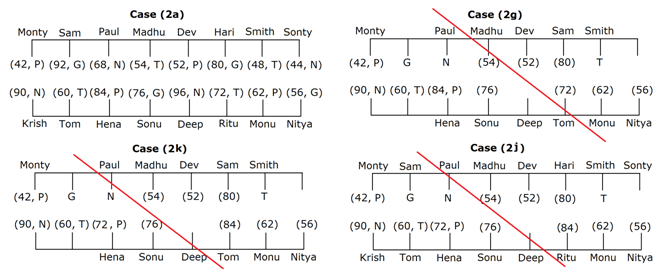Case (2g) & case (2k) are not valid as Hari sits second to right of Sonty and case (2j) is not valid as Ritu belongs to group T but her weight is not more than 75kg.

Clearly, the one whose weight is 44kg sits fourth to left of Madhu.

Hence, option B is correct choice.

Clearly, Sonu and Nityabelongs to group G from 6th class.

Hence, option A is correct choice.

Clearly, statement A and C are not true.

Hence, option E is correct choice.

Clearly, two person sits between Sonu and the one who sits facing Smith.

Hence, option A is correct choice.